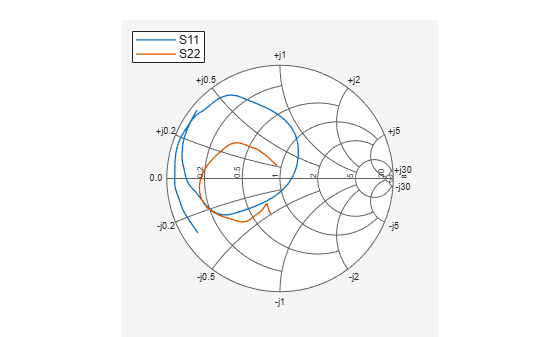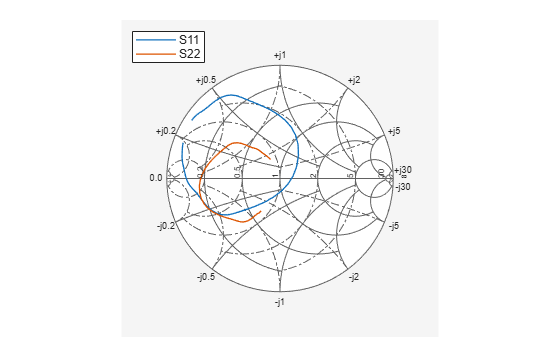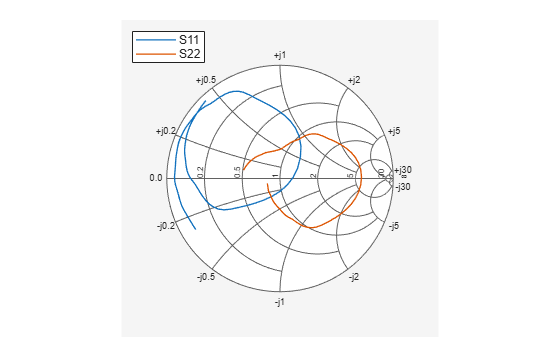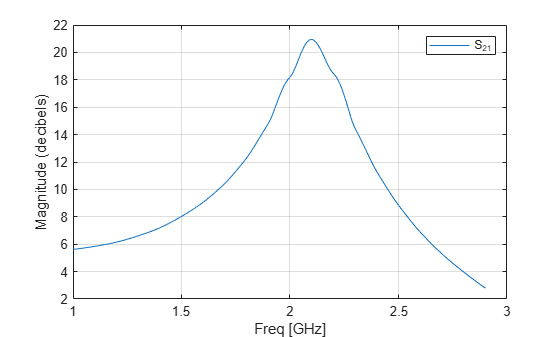Documentation

## RF Circuit Objects

This example shows how to create and use RF Toolbox™ circuit objects. In this example, you create three circuit (`rfckt`) objects: two transmission lines and an amplifier. You visualize the amplifier data using RF Toolbox™ functions and retrieve frequency data that was read from a file into the amplifier `rfckt` object. Then you analyze the amplifier over a different frequency range and visualize the results. Next, you cascade the three circuits to create a cascaded `rfckt` object. Then you analyze the cascaded network and visualize its S-parameters over the original frequency range of the amplifier. Finally, you plot the `S11`, `S22`, and `S21` parameters and noise figure of the cascaded network.

### Create `rfckt` Objects

Create three circuit objects: two transmission lines, and an amplifier using data from `default.amp` data file.

```FirstCkt = rfckt.txline; SecondCkt = rfckt.amplifier('IntpType','cubic'); read(SecondCkt,'default.amp'); ThirdCkt = rfckt.txline('LineLength',0.025,'PV',2.0e8); ```

### View Properties of `rfckt` Objects

You can use the `get` function to view an object's properties. For example,

```PropertiesOfFirstCkt = get(FirstCkt) ```
```PropertiesOfFirstCkt = struct with fields: LineLength: 0.0100 StubMode: 'NotAStub' Termination: 'NotApplicable' Freq: 1.0000e+09 Z0: 50.0000 + 0.0000i PV: 299792458 Loss: 0 IntpType: 'Linear' nPort: 2 AnalyzedResult: [] Name: 'Transmission Line' ```
```PropertiesOfSecondCkt = get(SecondCkt) ```
```PropertiesOfSecondCkt = struct with fields: NoiseData: [1x1 rfdata.noise] NonlinearData: [1x1 rfdata.power] IntpType: 'Cubic' NetworkData: [1x1 rfdata.network] nPort: 2 AnalyzedResult: [1x1 rfdata.data] Name: 'Amplifier' ```
```PropertiesOfThirdCkt = get(ThirdCkt) ```
```PropertiesOfThirdCkt = struct with fields: LineLength: 0.0250 StubMode: 'NotAStub' Termination: 'NotApplicable' Freq: 1.0000e+09 Z0: 50.0000 + 0.0000i PV: 200000000 Loss: 0 IntpType: 'Linear' nPort: 2 AnalyzedResult: [] Name: 'Transmission Line' ```

### List Methods of `rfckt` Objects

You can use the `methods` function to list an object's methods. For example,

```MethodsOfThirdCkt = methods(ThirdCkt); ```

### Change Properties of `rfckt` Objects

Use the `get` function or Dot Notation to get the line length of the first transmission line.

```DefaultLength = FirstCkt.LineLength; ```

Use the `set` function or Dot Notation to change the line length of the first transmission line.

```FirstCkt.LineLength = .001; NewLength = FirstCkt.LineLength; ```

### Plot the Amplifier S11 and S22 Parameters

Use the `smithplot` method of circuit object to plot the original `S11` and `S22` parameters of the amplifier (`SecondCkt`) on a Z Smith chart. The original frequencies of the amplifier's S-parameters range from 1.0 GHz to 2.9 GHz.

```figure smithplot(SecondCkt,[1 1;2 2]); ```### Plot the Amplifier Pin-Pout Data

Use the `plot` method of circuit object to plot the amplifier (`SecondCkt`) Pin-Pout data, in dBm, at 2.1 GHz on an X-Y plane.

```plot(SecondCkt,'Pout','dBm') legend('show','Location','northwest'); ```### Get the Original Frequency Data and the Result of the Analyzing the Amplifier over the Original Frequencies

When the RF Toolbox reads data from default.amp into an amplifier object (`SecondCkt`), it also analyzes the amplifier over the frequencies of network parameters in default.amp file and store the result at the property `AnalyzedResult`. Here are the original amplifier frequency and analyzed result over it.

```f = SecondCkt.AnalyzedResult.Freq; data = SecondCkt.AnalyzedResult ```
```data = rfdata.data with properties: Freq: [191x1 double] S_Parameters: [2x2x191 double] GroupDelay: [191x1 double] NF: [191x1 double] OIP3: [191x1 double] Z0: 50.0000 + 0.0000i ZS: 50.0000 + 0.0000i ZL: 50.0000 + 0.0000i IntpType: 'Cubic' Name: 'Data object' ```

### Analyze the Amplifier over a New Frequency Range and Plot Its New S11 and S22

To visualize the S-parameters of a circuit over a different frequency range, you must first analyze it over that frequency range.

```analyze(SecondCkt,1.85e9:1e7:2.55e9); smithplot(SecondCkt,[1 1;2 2],'GridType','ZY'); ```### Create and Analyze a Cascaded `rfckt` Object

Cascade three circuit objects to create a cascaded circuit object, and then analyze it at the original amplifier frequencies which range from 1.0 GHz to 2.9 GHz.

```CascadedCkt = rfckt.cascade('Ckts',{FirstCkt,SecondCkt,ThirdCkt}); analyze(CascadedCkt,f); ```Figure 1: The cascaded circuit.

### Plot the S11 and S22 Parameters of the Cascaded Circuit

Use the `smithplot` method of circuit object to plot `S11` and `S22` of the cascaded circuit (`CascadedCkt`) on a Z Smith chart.

```smithplot(CascadedCkt,[1 1;2 2],'GridType','Z'); ```### Plot the S21 Parameters of the Cascaded Circuit

Use the `plot` method of circuit object to plot `S21` of the cascaded circuit (`CascadedCkt`) on an X-Y plane.

```plot(CascadedCkt,'S21','dB') legend show ```### Plot the Budget S21 Parameters and Noise Figure of the Cascaded Circuit

Use the `plot` method of circuit object to plot the budget `S21` parameters and noise figure of the cascaded circuit (`CascadedCkt`) on an X-Y plane.

```plot(CascadedCkt,'budget','S21','NF') legend show ```